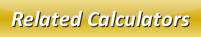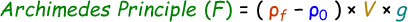# Archimedes Principle Calculator

Archimedes principle states when an object is immersed into the fluid is buoyed up by the force which is similar to the displaced fluid's weight.

The Archimedes principle can be applied for both submerged and floating objects in the fluid.

Formula to calculate Archimedes principle is given by:where,
F = Buoyant force of the body [N]
ρf = Object density [kg/m3]
ρ0 = Fluid density [kg/m3]
V = Volume of displaced fluid [m3]
g = Acceleration due to gravity [9.8 m/s2]

In our below online Archimedes principle calculator to calculate buoyant force of the body. Enter the input values and click calculate to find the output.

 Object Density (ρf): [kg/m3] Fluid Density (ρ0): [kg/m3] Volume of Displaced Fluid (V): [m3] Buoyant Force of the Body (F): [N]

Latest Calculator Release

Average Acceleration Calculator

Average acceleration is the object's change in speed for a specific given time period. ...

Free Fall Calculator

When an object falls into the ground due to planet's own gravitational force is known a...

Torque Calculator

Torque is nothing but a rotational force. In other words, the amount of force applied t...

Average Force Calculator

Average force can be explained as the amount of force exerted by the body moving at giv...

Angular Displacement Calculator

Angular displacement is the angle at which an object moves on a circular path. It is de...